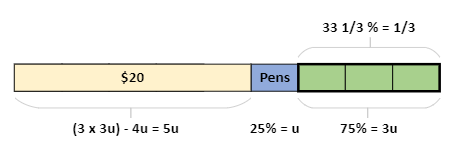# Question```Let the amount spent on pens be u (blue block).
So remaining amount after \$20 and pens is 3u (green blocks).
But that 3u is 33 1/3% = 1/3 of total amount.
So total = 3 x 3u = 9u and \$20 is represented as 9u - 4u = 5u.
Thus u = 4 and 9u = 36. He had \$36 at first.```
0 Replies 1 Like

Let u be the total amount of money Bala had at first.

Money Spent        Money Left

Books        20                          u-20

Pens         (1/4)(u-20)         (1/3)u  =  33 1/3 % of u  = [ (100/3) x (1/100)u = (1/3)u]

If money left at the end is (1/3)u, this means total money spent =  (2/3)u

20 + (1/4)(u-20) = (2/3)u       ( x 12 on both sides)

240 +3u – 60 = 8u

5u = 180

u = 36

Hence Bala had \$36 at first

0 Replies 1 Like